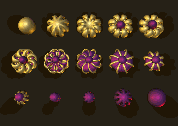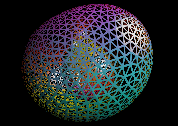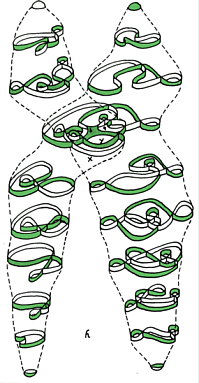Wednesday April 16

Discuss this week's assignment on coding.

Last Class: Started "topology of networks and surfaces":

Review Topology vocabulary:

"Bounded" In the plane- A line segment is bounded. A line is not bounded. A circle is bounded.  A parabola is not bounded. "Bounded" means the object can be visualized in a box.

"
closed
"  A line segment with endpoints is closed. A circle is closed.  A line is closed. A line segment missing one or both endpoints in not closed. A circle missing one point is not closed.

"
open
"  A line segment missing both endpoints is open. A line is open.  A circle missing one point is open.

"
connected
" A line, a line segment, a circle, a circle missing one point,  and a parabola are all connected.
A line segment missing an interior point is not connected.
It has two pieces.

The following two  letters  are topologically equivalent.

I   C

The following two  letters   are topologically equivalent to each other but not to the previous two letters. This can be seen by removing a single point from these letters which will not disconnect the curves, as it does with the previous letters.

O  D

The letter T missing the point where the top meets the vertical line segment is not connected. It has three pieces.

The letter Y missing the point where the top meets the vertical line segment also is not connected. It has three pieces.
T and Y are topologically  the same (equivalent)! this can be seen by bending the tops to the letters up or down and stretching and shrinking the lengths as well.

Questions: Is a triangle topologically equivalent to another triangle?
Yes. Stretch the sides and you'll also transform the angles.

Is an circle topologically equivalent to a line?  No.
The circle is still connected when you remove a point.
The line is disconnected when you remove a point.

Is a triangle topologically equivalent to a square? a circle? yes.

Maps
Coordinates for "earth" - the sphere
Coordinates for the torus!

Activity for maps on Torus.
Locate P and Q on the map! give their coordinates.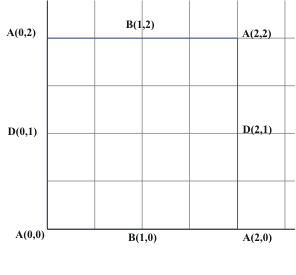Topology and measurements:

• Counting on a line.  Counting on a curve.
Keep count on the number of vertices and segments for a graph.   Compare:

 Vertices Edges V-E Line segment I 2 1 1 circle O 1 1 0 8 1 2 -1 9 2 2 0 B 2 3 -1

Curves will not be topologically equivalent if the number V-E is not the same.

However, as the table shows, there are curves that have the same number V-E which are also not equivalent.

These can be distinguished by other criterion, such as connectivity when a point is removed.

The
number V-E is a characteristic of the curve
that will be the same for any topologically equivalent curve.

However, this number does not completely classify the curves topologically, since curves can have the same number V-E without being equivalent.

Counting in the plane or Counting on a surface.
Vertices, edges, regions.

 Vertices Regions Edges Line segment 2 1 1 circle 1 2 1 8 1 3 2 9 2 2 2 B 2 3 3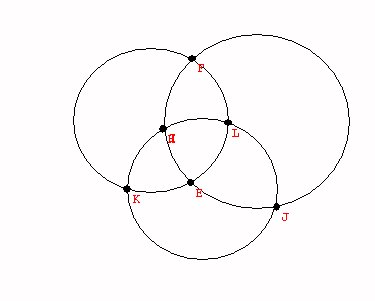6 8 12

Counting in the plane or Counting on a sphere.

Euler's Formula for the plane or the sphere:
Theorem: For any connected graph in the plane,

V+R = E + 2.
OR  V -E+R =2

The number 2 is called the "Euler characteristic for the plane."  ( and the sphere).

Proof:  Deconstruct the graph.
First remove edges to "connect regions" until there is only one region.  The relation between V+R and E+ 2 stays the same.  (One edge goes with one region.)

Now remove one vertex and edge to "trim the tree".
Again the relation between V+R and E+2 stays the same. ( One edge goes with each vertex.)

Finally we have just one vertex, no edges, and one region.
So the relationship is that V+R = E + 2!

For any connected graph on the sphere, V+R = E + 2

Proof:
Choose a point P on the sphere not on the graph.
Place a plane touching the sphere opposite to P.
Then project the graph onto the plane.

The projected graph will have the same number of vertices, edges and regions.

The counting in the plane shows that the formula is true for the projected graph, and thus for the original graph on the sphere!

1. What about graphs on the Torus?
We have a connected graph on the torus with one vertex, two edges and only on region. But this information does not match for the euler formula for the plane or the sphere. SO.. the torus must be topologically different from the sphere or the plane!
In fact for the torus we see that it is possible for V+R= E   or  V-E+R= 0

Summary: We have learned about (and proved) Euler's Formula for the plane or the sphere:

For any connected graph in the plane or on the sphere, V+R = E + 2.
Adventures on the Mobius Band, the Klein Bottle, and the Projective Plane !
"New" Surfaces and
The Classification of Surfaces

What is a surface?
Bounded, unbounded:
Closed, open:

With or without boundary:
Orientable or Non-orientable:
The Moebius band is a
non-orientable surface.
Introduce the Klein Bottle and Projective Plane as examples of non-orientable surfaces.
A surface can be realized (imbedded) in a plane, in 3 space, in 4 space.
A surface can be visualized (immersed) in ...

Review examples: A closed disc, an open disc, a plane, an annulus- cylinder, a mobius band;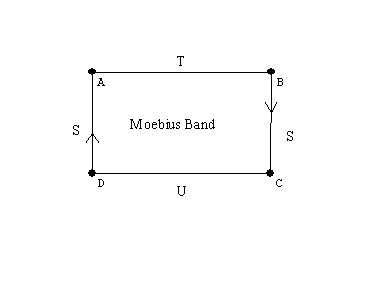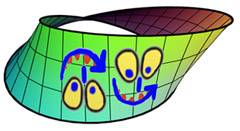Mirror image and orientability - see the animated version (73K) Wind and Mr. Ug The Möbius band is one-sided - view the animated version (1.3M)

Experiments with the mobius band:
Draw a curve along the center of the band- we cover both "sides."
Cut along that curve- the band does not fall apart, but gets twice as long!

A sphere

A torus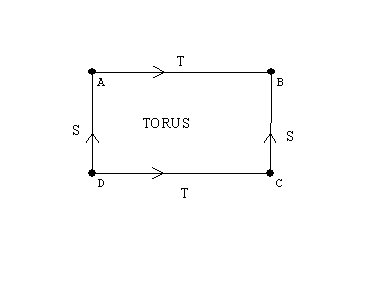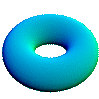[Activity:Graphs on the torus]

A Klein bottle:# A javaview visualization of the Klein bottle

On Line Article: Imaging maths - Inside the Klein bottle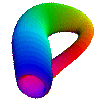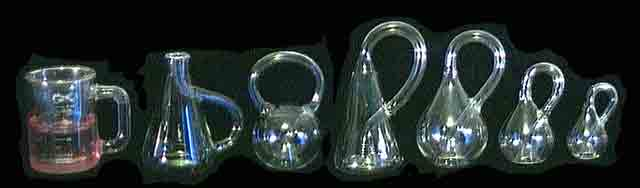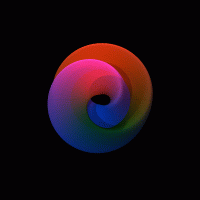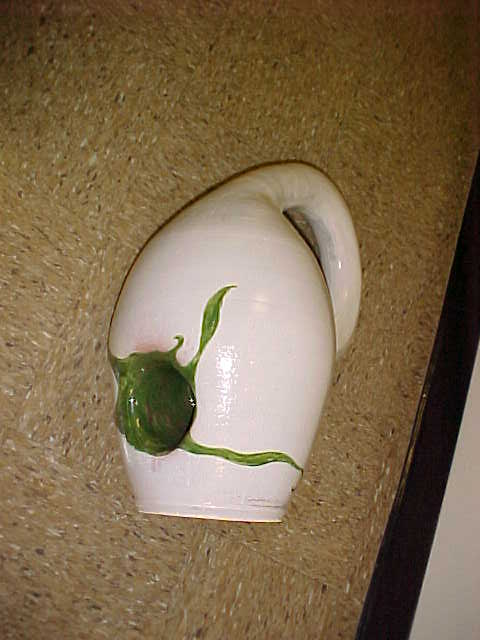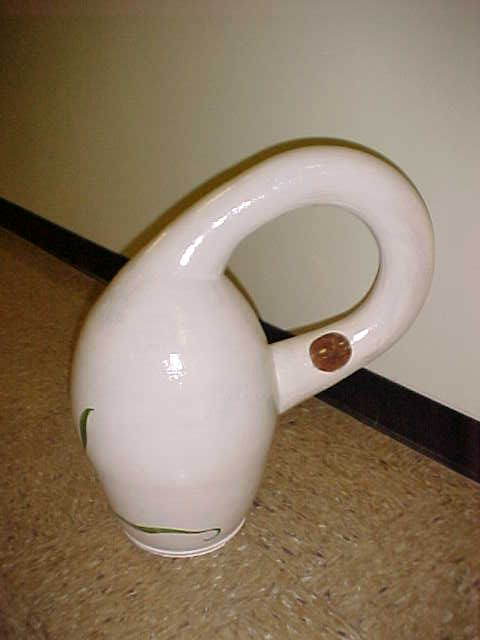The projective plane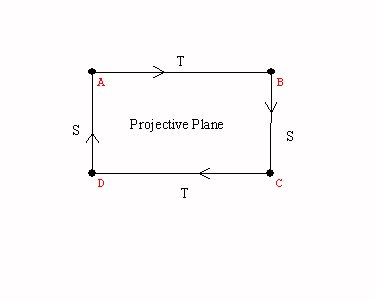Why is this a closed and bounded surface?

Spheres with handles: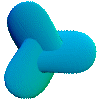Spheres with cross caps

Visualizations of surfaces by flattened - cut apart models.
A cylinder, a mobius band, the torus, the Klein bottle, the projective plane.

Handles and cross-caps  attached to the sphere.

The Topological Classification of "closed surfaces.

" If Mathematicians Made Pretzels" Proof without words.
The following two figures are topologically equivalent.

From the Fun Fact files, hosted by the Harvey Mudd College Math Department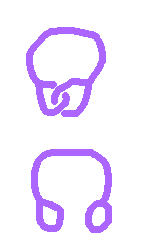Figure 1Figure 2

Imagine that the two objects in Figure 1 are solid (with thickness) and made of very flexible and stretchy rubber.

Question: is it possible to deform one object into the other in a continuous motion (without tearing or cutting)?

Hint: it is important that the object is solid and has thickness; this transformation cannot be done with a one-dimensional piece of string. It is also not possible to do this with a piece of rope because even though the rope has thickness, it is not flexible or "stretchy" enough. See below for an explanation and animated gif. Or, don't scroll down if you want to think about it a while!

The Math Behind the Fact:
One way to do this is the following. Widen one of the loops and move one of its handles along the stem between the two loops to the other loop and push it through the hole so that the two loops become unlinked. The reference contains a sequence of pictures of this transformation.

Graeme McRae has generously contributed the animated gif in Figure 2, showing another solution to this problem! (Thank you, Graeme!)
Here is the original picture:Ways to think of surfaces : cross-sections/ projections/moving curves/ using color to see another dimension.
How do 3d glasses work?
Spheres with handles,

Visualizations of surfaces by flattened - cut apart models.
A cylinder, a mobius band, the torus, the Klein bottle, the projective plane.

Closed Surfaces: Handles and cross-caps  attached to the sphere.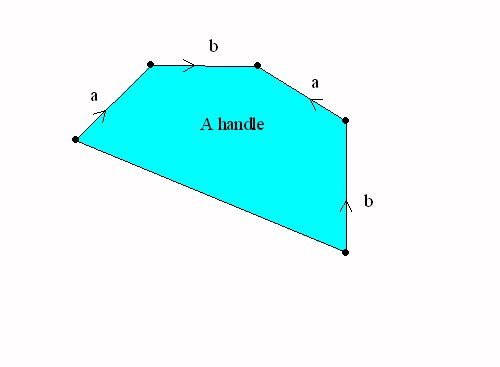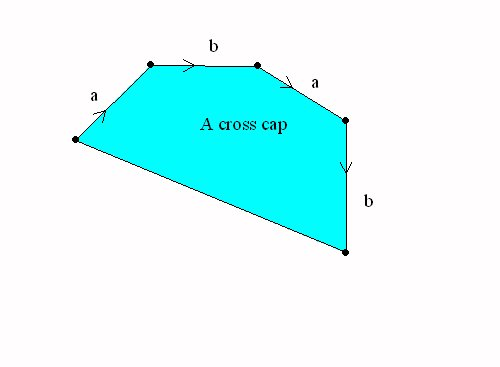A sphere with a handle = a torus A Sphere with a cross cap = the projective plane

The Topological Classification of "closed surfaces."

Every connected closed and bounded surface is topologically equivalent to a sphere with handles and crosscaps attached.

The Euler Characteristic of A Surface.

The classification of surfaces determines the euler characteristic of each surface.
If the surface is orientable, it is a sphere with n handles, so V-E+R = 1 - 2n +1 = 2-2n.  For example the torus has euler characteristic 2-2*1=0.
If the surface is non-orientable, then is it a sphere with k crosscaps and n handles, so the euler characteristic is V-E+R = 1 - (k+2n) +1 =2 -2n -k.
Notice that a sphere with two cross caps has euler characteristic 0, the same as the torus. But this was the euler characteristic of the Klein Bottle. So we should be able to recognize the Klein bottle as a sphere with two cross caps.
This can be done by a single normalization of one pair of edges with the same  orientation.

Other interest in surfaces: Examples
Ways to think of surfaces : cross-sections/ projections/moving curves/ using color to see another dimension.

Generalization of surfaces are called "manifolds".  cross sections / projections/ moving surfaces-solids.
"minimal surfaces" (FAPP video?)
Transforming surfaces: "turning the sphere inside out." video